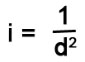# Definition: Inverse square law#### Definition: Inverse square law

##### The inverse square law – light dims with distance

As light radiates away from a light source it loses its intensity. The same overall energy is still present but the light has more area to illuminate. It turns out that there is a direct mathematical relationship.

In the diagram you can see how the light spreads out. At a distance of one unit from the source the size of the square area the light illuminates is equal to 1 square unit. At a distance of two units from the source the size of the area the light has spread over is twice the size. So the area the light is spread over is the distance squared.

This relationship continues…

1 unit distance from source the square is 1 x 1 (1 sq).
2 units distance from source the square is 2 x 2 (4 sq).
3 units distance from source the square is 3 x 3 (9 sq).
4 units distance from source the square is 4 x 4 (16 sq).
…and so on.

Note:
For simplicity the 3 x 3 square is not shown in the diagram.

Each time the distance increases, the illuminated area also gets squared larger. The same amount of energy is spread out more and more. The diagram shows the distance (d) at four units from the source. 4² = 16 squares. Now the total light that illuminated the first square is spread over 16 times the area (4 squares by 4 squares). So the light in any one square is 1/16th of the total light.

This “inverse square law” can be expressed in a simple equation. Where it applies to light it says:Where:
i = Intensity of light
d = distance units from the source (normally meters)

In the case where d = 4 units (shown in the diagram) we can say: i = 1/4² or i = 1/16th. In every day words we can say, “the light intensity at four units from source is one sixteenth of the intensity at one unit distance from the source”.

When we describe this verbally in maths we say, “the intensity of light varies with the inverse of the square of the distance from the source”.

##### Why learn about the inverse square law?

It is useful to know about this relationship. Each time we set up a scene to make an image we are playing with the properties of light. The Inverse Square Law tells us how the light will dim as we put lights further away from the subject. Each time we move it one unit of distance further away the light will dim by the square of the distance. That means a big reduction in light each time we move the light further back. We need to factor that reduction into how we set up lights.

[refdef?][refdef?][refdef?]#????#Damon Guy (Netkonnexion)

Damon is a writer-photographer and editor of this site. He has also run some major websites, a computing department and a digital image library. He started out as a trained teacher and now runs training for digital photogs.Photokonnexion tips by email